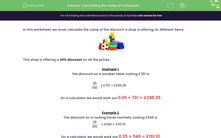# Calculating the Value of a Discount

In this worksheet, students calculate the actual discount on different items given a percentage discount. Calculators may be used.Key stage:  KS 2

Curriculum topic:   Number: Fractions, Decimals and Percentages

Curriculum subtopic:   Know Fraction-Decimal-Percentage Equivalents

Popular topics:   Money worksheets

Difficulty level:#### Worksheet Overview

In this worksheet, we must calculate the value of the discount a shop is offering on different items.This shop is offering a 35% discount on all the prices.

Example 1

The discount on a snooker table costing £701 is

 35 100
x £701 = £245.35

On a calculator we would work out 0.35 × 701 = £245.35

Example 2

The discount on a rocking horse normally costing £546 is

 35 100
x £546 = £191.10

On a calculator we would work out 0.35 × 546 = £191.10

### What is EdPlace?

We're your National Curriculum aligned online education content provider helping each child succeed in English, maths and science from year 1 to GCSE. With an EdPlace account you’ll be able to track and measure progress, helping each child achieve their best. We build confidence and attainment by personalising each child’s learning at a level that suits them.

Get started